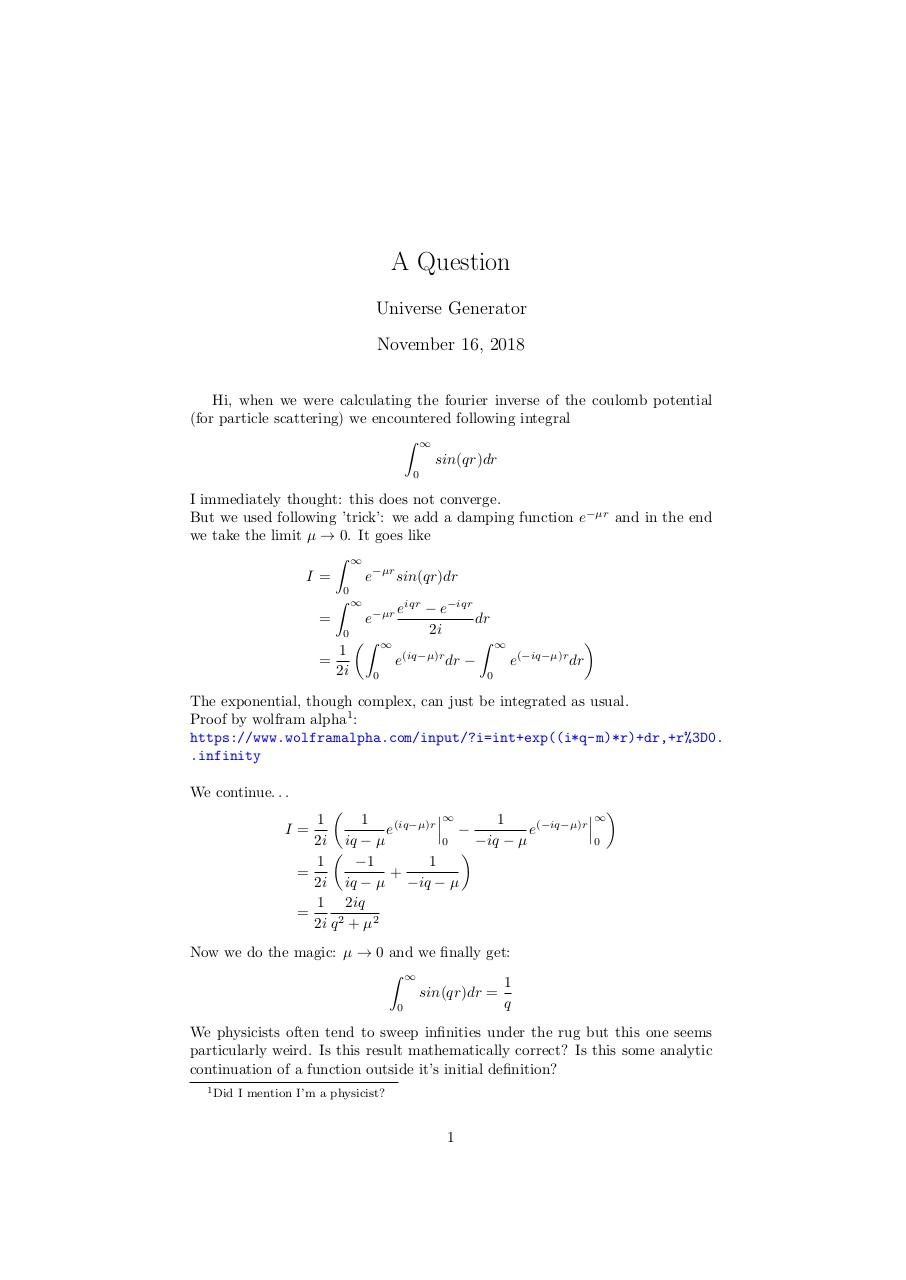# sinqr .pdf

### File information

Original filename: sinqr.pdf

This PDF 1.5 document has been generated by LaTeX with hyperref package / pdfTeX-1.40.19, and has been sent on pdf-archive.com on 16/11/2018 at 23:42, from IP address 213.49.x.x. The current document download page has been viewed 850 times.
File size: 125 KB (1 page).
Privacy: public file

sinqr.pdf (PDF, 125 KB)

### Document preview

A Question
Universe Generator
November 16, 2018
Hi, when we were calculating the fourier inverse of the coulomb potential
(for particle scattering) we encountered following integral
Z ∞
sin(qr)dr
0

I immediately thought: this does not converge.
But we used following ’trick’: we add a damping function e−µr and in the end
we take the limit µ → 0. It goes like
Z ∞
I=
e−µr sin(qr)dr
0
Z ∞
eiqr − e−iqr
dr
=
e−µr
2i
0
Z ∞

Z ∞
1
(iq−µ)r
(−iq−µ)r
=
e
dr −
e
dr
2i
0
0
The exponential, though complex, can just be integrated as usual.
Proof by wolfram alpha1 :
https://www.wolframalpha.com/input/?i=int+exp((i*q-m)*r)+dr,+r%3D0.
.infinity
We continue. . .


∞ 
1
1
1
(iq−µ)r
(−iq−µ)r
I=
e
e

2i iq − µ
−iq − µ
0
0


1
−1
1
=
+
2i iq − µ −iq − µ
1 2iq
=
2i q 2 + µ2
Now we do the magic: µ → 0 and we finally get:
Z ∞
1
sin(qr)dr =
q
0
We physicists often tend to sweep infinities under the rug but this one seems
particularly weird. Is this result mathematically correct? Is this some analytic
continuation of a function outside it’s initial definition?
1 Did

I mention I’m a physicist?

1#### HTML Code

Copy the following HTML code to share your document on a Website or Blog

#### QR Code### Related keywords